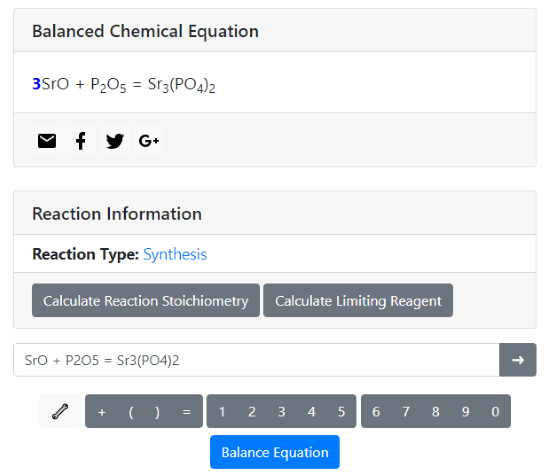Editor Ratings:
User Ratings:
[Total: 0 Average: 0]

Balancing chemical equations is not always easy. There are very complex chemical equations which can take a significant amount of time to balance. Having a tool to balance chemical equation automatically can be very useful in such scenario. A chemical equation balancer can be used by professionals to save time and by students to get help in their studies.

With the help of these websites, you can easily balance chemical equations in a matter of seconds. All you have to do is enter your chemical equation on these websites and they will balance it for you and highlight the changes. Some of these websites also give you additional information such as reaction type, reaction thermodynamics, etc. You can also calculate the Reaction Stoichiometry and Limiting Reagent as well. So, let’s check out these websites one by one.

Also read: Free Chemical Simulator Software To Simulate Chemical Reactions

## WolframAlpha.comWolframAlpha is a multi-function online dynamic computation tool where you can perform calculations on various types of data. Educational data and other day-to-day tasks are the main focus here including Mathematics, Science & Technology, Society & Culture, and Everyday Life. With WolframAlpha’s Chemical Equation Balancing tool, you can quickly find the correct coefficients for your chemical reaction.

Here, you can input the chemical reaction in three different ways. You can either enter the formulae(symbols) of chemical compounds, or you can type their full name instead, or you can mix them both. This way, you can easily input compounds like octane, glucose, etc. without the need of their formulae. When you parse your chemical equation through this tool, its detects your input and shows its interpretation. Below that, it gives you a step by step solution to balance the equation and shows their structures as well.

Moreover, WolframAlpha also calculates the Reaction Thermodynamics and gives you its EnthalpyEntropy, and Equilibrium constant. And, if you upgrade to WolframAlpha Pro, you can download all these results and unlock many other features like image input, data input, file upload, etc.

You can use this online chemical equation balancer here.

## WebQC.orgWebQC.org is an online chemical portal with Chemistry Tools, Unit Converters, Chemical Symmetry, and more. Under the Chemistry Tools, it has a Chemical Equation Balancer which you can use to balance your chemical reactions online. Just like WolframAlpha, you can use names, symbols or/and formulae to input your chemical equation here. In the results, it gives you a perfectly balanced chemical equation with color-coded new entries. Apart from that, this website shows you a table of the chemical compounds of your equation with their Coefficients and Molar Mass. With this table, you can calculate the Reaction Stoichiometry and Limiting Reagent. All you have to do is enter the number of moles of compounds and the table will show you its weight.

Give this online chemical equation balancer a try here.

## ChemicalAid.comChemicalAid is another free website that provides information on chemistry and chemical elements. This website has a Chemical Equation Balancer that balances the chemical equations and also determines their reaction types. You can directly input your equation in this tool either by using chemical names or formulae, or both. It also has a periodic table from which you can use to choose the elements for the reactions.

In the results, this tool balances the chemical reaction for you and highlights the changes. It also tells you whether the reaction is Combustion, SynthesisDecompositionSingle displacementDouble displacement, or Acid-Base type. With this tool, you can easily calculate the Reaction Stoichiometry and Limiting Reagent as well.

Here is the link to try this online chemical equation balancer.

## TheodoreGrey.comThe next chemical equation balancer tool on this list is from TheodoreGrey.com. This is a simple and straightforward chemical equation balancer that takes the input only in chemical symbols. To balance your equation, type it here and click the “Calculate button”. This tool gives you the balanced reaction in the results. It also gives you the balanced weight of the reagents. You can enter a custom weight for a regent and it calculates the weight of other reagents for the reaction. This feature comes handy for chemistry experiments when you have to carry a reaction for a custom amount.

This tool also gives you the molecular weight of each reagent along with their weight-percentage composition. And, you can also calculate Limiting Reagents for a reaction.

You can try this online chemical equation balancer here.

## EndMemo.comEndMemo is a free website that packs a variety of educational tools and calculators covering Mathematics, Physics, Chemistry, Biology, Literature, Health, and more. It has a simple chemical equation balancer that can help you balance an unbalanced chemical equation. To do that, you can either use the periodic table to make an equation or directly enter an equation in the given text field using chemical symbols. After entering the equation, you can check whether it’s already balanced or not using the “Check” button. In the case of an unbalanced equation, click the “Balance” button to balance it. Once you do that, it balances the equation and shows you the result below the input text field. It highlights the changes it made to the equation so that you can easily notice how it balanced the equation.

Try this online chemical equation balancer here.

### Closing Words:

These are the 5 free online chemical equation balancer websites. You can quickly balance chemical equations using any of these websites. Wolfram Alpha gives you lots of other information but it does take some time to calculate all that information. So, if you just need to balance the equation, I recommend you to use others, but if you need details, then, Wolfram Alpha is the one to go with.

 Editor Ratings: User Ratings:[Total: 0 Average: 0] Free/Paid: Free Tags: chemistryequation